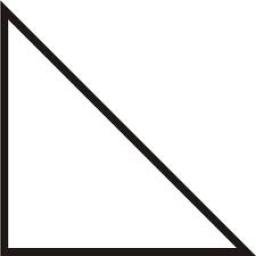# Right triangle

Legs of the right triangle are in the ratio a:b = 2:8. The hypotenuse has a length of 87 cm.

Calculate the perimeter and area of the triangle.

Result

o =  192.503 cm
S =  890.471 cm2

#### Solution:

$c = 87 \ cm \ \\ a:b = 2:8 \ \\ c^2 = a^2+b^2 \ \\ c^2 = a^2+(8/2 \cdot \ a)^2 \ \\ c^2 = a^2+(8/2)^2 \ a^2 \ \\ c^2 = a^2 (1 +(8/2)^2 ) \ \\ \ \\ a = c / \sqrt{ 1 +(8/2)^2 } = 87 / \sqrt{ 1 +(8/2)^2 } \doteq 21.1006 \ cm \ \\ \ \\ b = a \cdot \ \dfrac{ 8 }{ 2 } = 21.1006 \cdot \ \dfrac{ 8 }{ 2 } \doteq 84.4024 \ cm \ \\ \ \\ o = a+b+c = 21.1006+84.4024+87 \doteq 192.503 = 192.503 \ \text{ cm }$
$S = \dfrac{ a \cdot \ b }{ 2 } = \dfrac{ 21.1006 \cdot \ 84.4024 }{ 2 } = \dfrac{ 15138 }{ 17 } \doteq 890.4706 = 890.471 \ cm^2$

Try calculation via our triangle calculator.Our examples were largely sent or created by pupils and students themselves. Therefore, we would be pleased if you could send us any errors you found, spelling mistakes, or rephasing the example. Thank you!

Leave us a comment of this math problem and its solution (i.e. if it is still somewhat unclear...):Be the first to comment!Tips to related online calculators
Looking for help with calculating roots of a quadratic equation?
Check out our ratio calculator.
Do you have a linear equation or system of equations and looking for its solution? Or do you have quadratic equation?
Pythagorean theorem is the base for the right triangle calculator.

## Next similar math problems:

1. Isosceles triangleThe perimeter of an isosceles triangle is 112 cm. The length of the arm to the length of the base is at ratio 5:6. Find the triangle area.
2. Isosceles trapezoidCalculate the content of an isosceles trapezoid whose bases are at ratio 5:3, the arm is 6cm long and it is 4cm high.
3. Sides of right angled triangleOne leg is 1 m shorter than the hypotenuse, and the second leg is 2 m shorter than the hypotenuse. Find the lengths of all sides of the right-angled triangle.
4. Three parallelsThe vertices of an equilateral triangle lie on 3 different parallel lines. The middle line is 5 m and 3 m distant from the end lines. Calculate the height of this triangle.
5. Two chordsCalculate the length of chord AB and perpendicular chord BC to circle if AB is 4 cm from the center of the circle and BC 8 cm from the center of the circle.
6. Equilateral triangleA square is inscribed into an equilateral triangle with a side of 10 cm. Calculate the length of the square side.
7. AP RT triangleThe length of the sides of a right triangle form an arithmetic progression, longer leg is 24 cm long. What are the perimeter and area?
8. Solid cuboidA solid cuboid has a volume of 40 cm3. The cuboid has a total surface area of 100 cm squared. One edge of the cuboid has length 2 cm. Find the length of a diagonal of the cuboid. Give your answer correct to 3 sig. Fig.
9. Diagonal 20Diagonal pathway for the rectangular town plaza whose length is 20 m longer than the width. if the pathway is 20 m shorter than twice the width. How long should the pathway be?
10. Right triangle eq2Find the lengths of the sides and the angles in the right triangle. Given area S = 210 and perimeter o = 70.
11. Three points 2The three points A(3, 8), B(6, 2) and C(10, 2). The point D is such that the line DA is perpendicular to AB and DC is parallel to AB. Calculate the coordinates of D.
12. Nice prismCalculate the surface of the cuboid if the sum of its edges is a + b + c = 19 cm and the body diagonal size u = 13 cm.
13. Rectangular triangleThe lengths of the rectangular triangle sides with a longer leg 12 cm form an arithmetic sequence. What is the area of the triangle?
14. Faces diagonalsIf the diagonals of a cuboid are x, y, and z (wall diagonals or three faces) respectively than find the volume of a cuboid. Solve for x=1.2, y=1.7, z=1.45
15. Touch x-axisFind the equations of circles that pass through points A (-2; 4) and B (0; 2) and touch the x-axis.
16. Sphere from tree pointsEquation of sphere with three point (a,0,0), (0, a,0), (0,0, a) and center lies on plane x+y+z=a
17. A bridgeA bridge over a river is in the shape of the arc of a circle with each base of the bridge at the river's edge. At the center of the river, the bridge is 10 feet above the water. At 27 feet from the edge of the river, the bridge is 9 feet above the water.